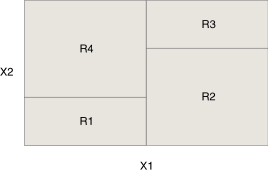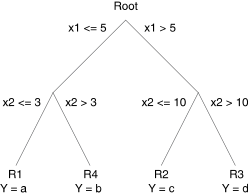# 1.0 - 8.00 - Introduction to Decision Trees - Teradata Vantage

## Teradata® Vantage Machine Learning Engine Analytic Function Reference

Product
Release Number
1.0
8.00
Release Date
May 2019
Content Type
Programming Reference
Publication ID
B700-4003-098K
Language
English (United States)

Decision trees are a supervised learning technique used for both classification and regression problems. A decision tree creates a piecewise constant approximation function for the training data. Decision trees are used in data mining and supervised learning because they are robust to many problems with real world data, such as missing values, irrelevant variables, outliers in input variables, and differences in variable scales.

The single decision tree algorithm, implemented in the Decision Tree Functions, is easy to use and has few variables to tune. However, it is prone to over-fitting and high variance. To help address this issue, the ML Engine provides the Decision Forest Functions, AdaBoost Functions, and XGBoost Functions. These functions create many trees from the same data set and combine the results to reduce the variance and the risk of over-fitting.

## Decision Tree Basics

Suppose that you want to predict the value of a variable, y, and you have two predictor variables, x1 and x2. You want to model y as a function of x1 and x2 (y = f(x1, x2)).

You can visualize x1 and x2 as forming a plane, and values of y at particular coordinates of (x1, x2) rising from the plane in the third dimension. A decision tree partitions the plane into rectangles and assigns each partition to predict a constant value of y, which is usually the average value of all the y values in that region. You can extend this two-dimensional example into arbitrarily many dimensions to fit models with large numbers of predictors.In this example, the x1-x2 plane has four regions, R1, R2, R3 and R4. The predicted value of y for any test observation in R1 is the average value of y for all training observations in R1.

This information can be represented by a decision tree:The algorithm starts at the Root node. If the x1 value for a data point is greater than 5, the algorithm follows the right path; if the value of x1 is less than 5, the algorithm follows the left path. At each subsequent node, the algorithm determines which branch to follow, until it reaches a leaf node, to which it assigns a prediction value.

• Decision trees are easy to visualize and understand.
• Decision trees offer an interpretable reason for their decisions.

For example: Person X is high-risk because his income is below C, his total debt is above D, and his age is below A.

• Decision trees are robust to spurious, co-linear, and correlated input variables.

• Decision tree training is a highly unstable procedure.

Small differences in the training set can cause very different decision tree structures, and often very different outcomes.

• Because they are piecewise-constant approximations, observations on regional boundaries are prone to high error rates.

## Boosting and Decision Trees

Boosting is a technique that develops a strong classifying algorithm from a collection of weak classifying algorithms. A classifying algorithm is weak if its correct classification rate is slightly better than random guessing (which is 50% for binary classification). The intuition behind boosting is that combining a set of predictions, each of which has more than 50% probability of being correct, can produce an arbitrarily accurate predictor function.

Without boosting, a decision tree has these inherent problems:
• Its growth is based on binary splitting, which can introduce inaccuracy in classification.
• An incorrect decision at one tree level propagates to the next level.

Boosting is sensitive to noise in the data. Because weak classifiers are likely to incorrectly classify outliers, the algorithm weights outliers more heavily with each iteration, thereby increasing their influence on the final result.